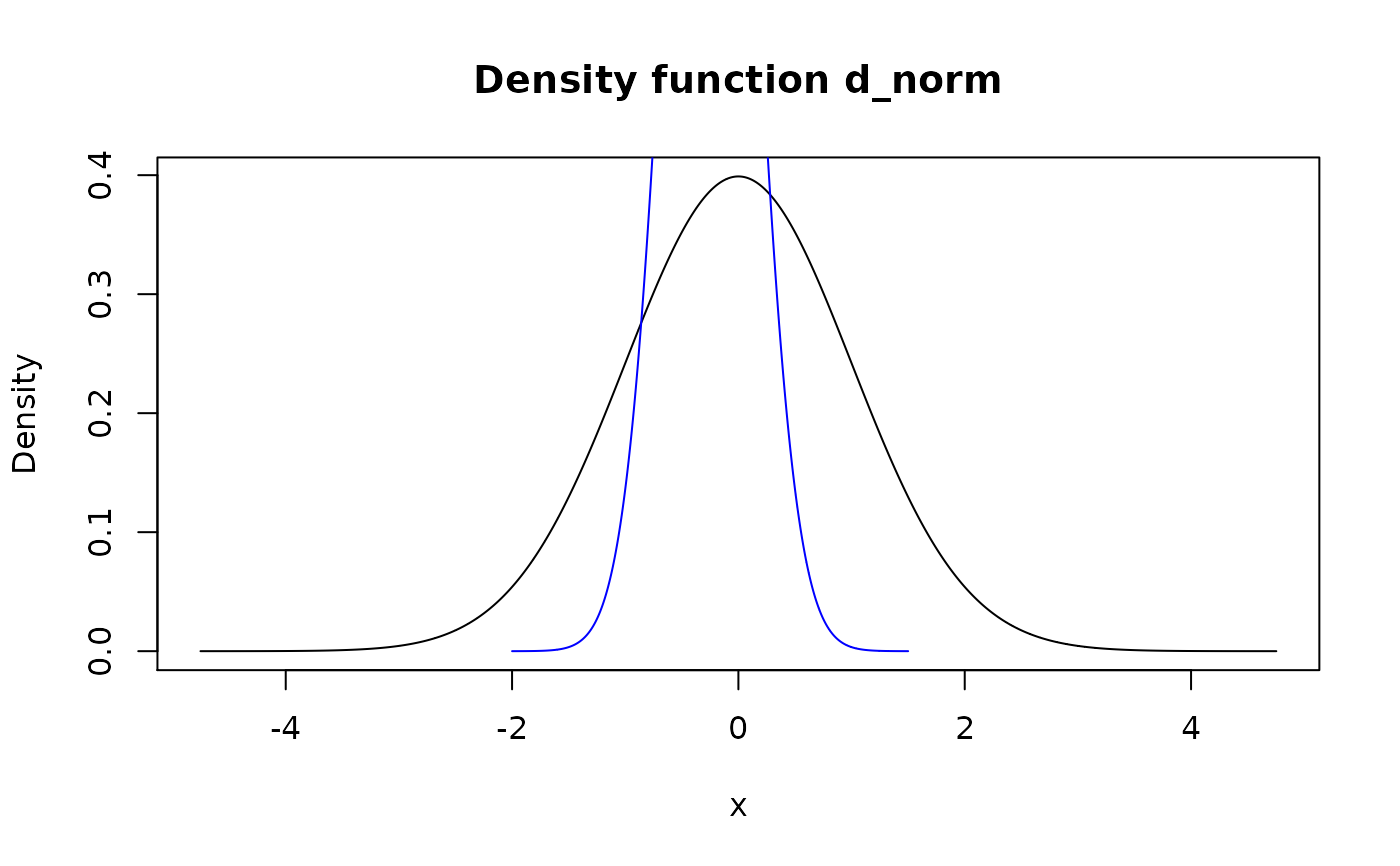Modify support of pdqr-function using method of choice.

form_resupport(f, support, method = "reflect")

Arguments

f A pdqr-function. Numeric vector with two increasing (or non-decreasing, see Details) elements describing support of the output. Values can be NA, in which case corresponding edge(s) are taken from f's support. Resupport method. One of "reflect", "trim", "winsor", "linear".

Value

A pdqr-function with modified support and the same class and type as f.

Details

Method "reflect" takes a density "tails" to the left of support and to the right of support and reflects them inside support. It means that values of density inside and outside of supplied support are added together in "symmetric fashion": d(x) = d_f(x) + d_f(l - (x-l)) + d_f(r + (r-x)), where d_f is density of input, d is density of output, l and r are left and right edges of input support. This option is useful for repairing support of new_*()'s output, as by default kernel density estimation in density() adds tails to the range of input x values. For example, if there is a need to ensure that distribution has only positive values, one can do form_resupport(f, c(0, NA), method = "reflect"). Notes:

• For "discrete" pdqr-functions that might result into creating new "x" values of distribution.

• Reflection over support is done only if it is strictly greater than f's left edge of support. Reflection over support - if f's right edge is strictly smaller.

Method "trim" removes density "tails" outside of support, normalizes the rest and creates appropriate pdqr-function.

Method "winsor" makes all density "tails" outside of input support "squashed" inside it in "dirac-like" fashion. It means that probability from both tails is moved inside support and becomes concentrated in 1e-8 neighborhood of nearest edge. This models a singular dirac distributions at the edges of support. Note that support can represent single point, in which case output has single element if f's type is "discrete" or is a dirac-like distribution in case of "continuous" type.

Method "linear" transforms f's support linearly to be input support. For example, if f's support is [0; 1] and support is c(-1, 1), linear resupport is equivalent to 2*f - 1. Note that support can represent single point with the same effect as in "winsor" method.

form_regrid() for changing grid (rows of "x_tbl" metadata) of pdqr-function.

form_retype() for changing type of pdqr-function.

Other form functions: form_estimate(), form_mix(), form_regrid(), form_retype(), form_smooth(), form_tails(), form_trans()

Examples

set.seed(101) d_norm <- as_d(dnorm) d_dis <- new_d(data.frame(x = 1:4, prob = 1:4 / 10), "discrete") # Method "reflect" plot(d_norm)
lines(form_resupport(d_norm, c(-2, 1.5), "reflect"), col = "blue")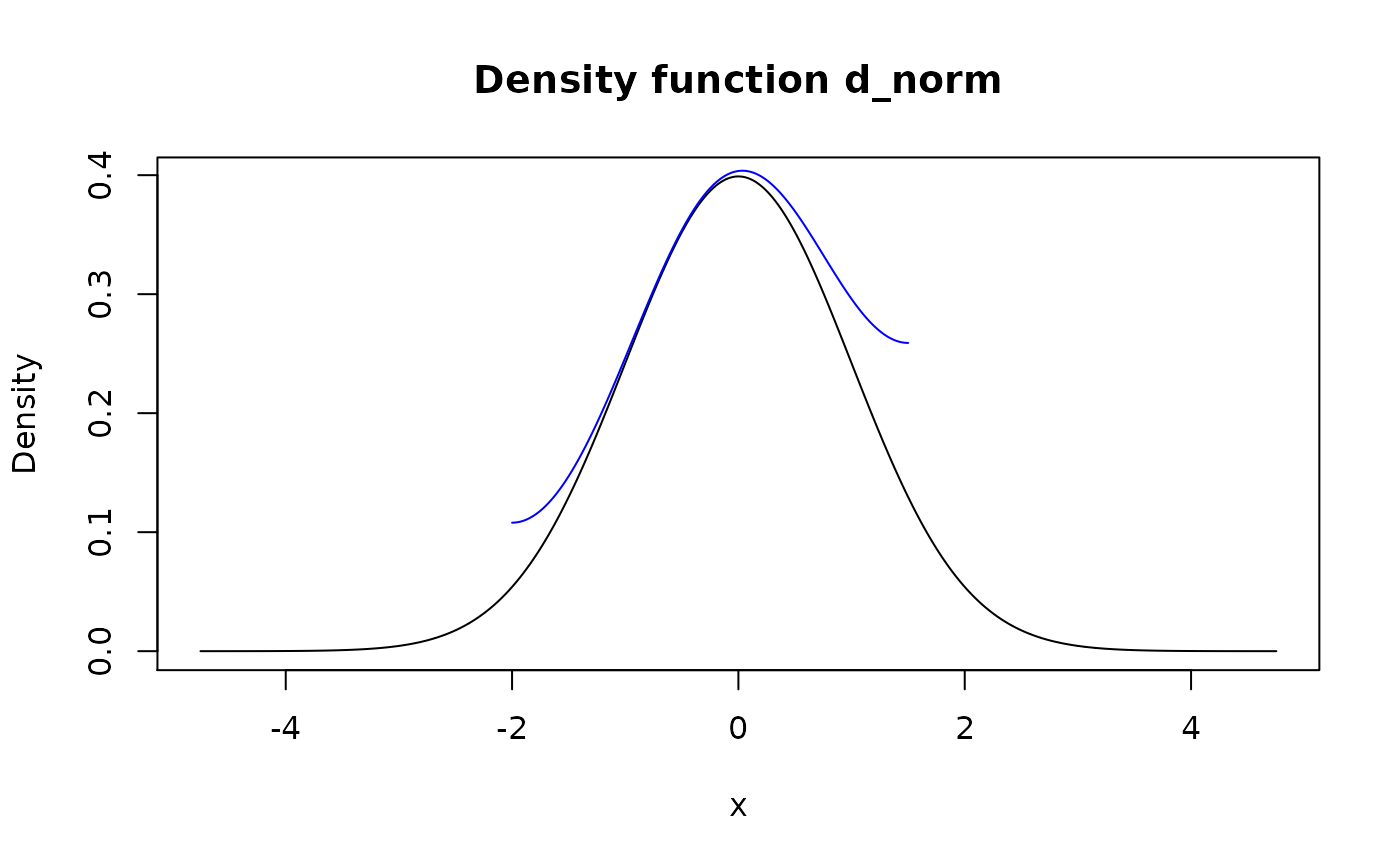# For "discrete" functions it might create new values meta_x_tbl(form_resupport(d_dis, c(NA, 2.25), "reflect"))
#> x prob cumprob #> 1 1.0 0.1666667 0.1666667 #> 2 1.5 0.5000000 0.6666667 #> 3 2.0 0.3333333 1.0000000
# This is often useful to ensure constraints after new_() x <- runif(1e4) d_x <- new_d(x, "continuous") plot(d_x)
lines(form_resupport(d_x, c(0, NA), "reflect"), col = "red")
lines(form_resupport(d_x, c(0, 1), "reflect"), col = "blue")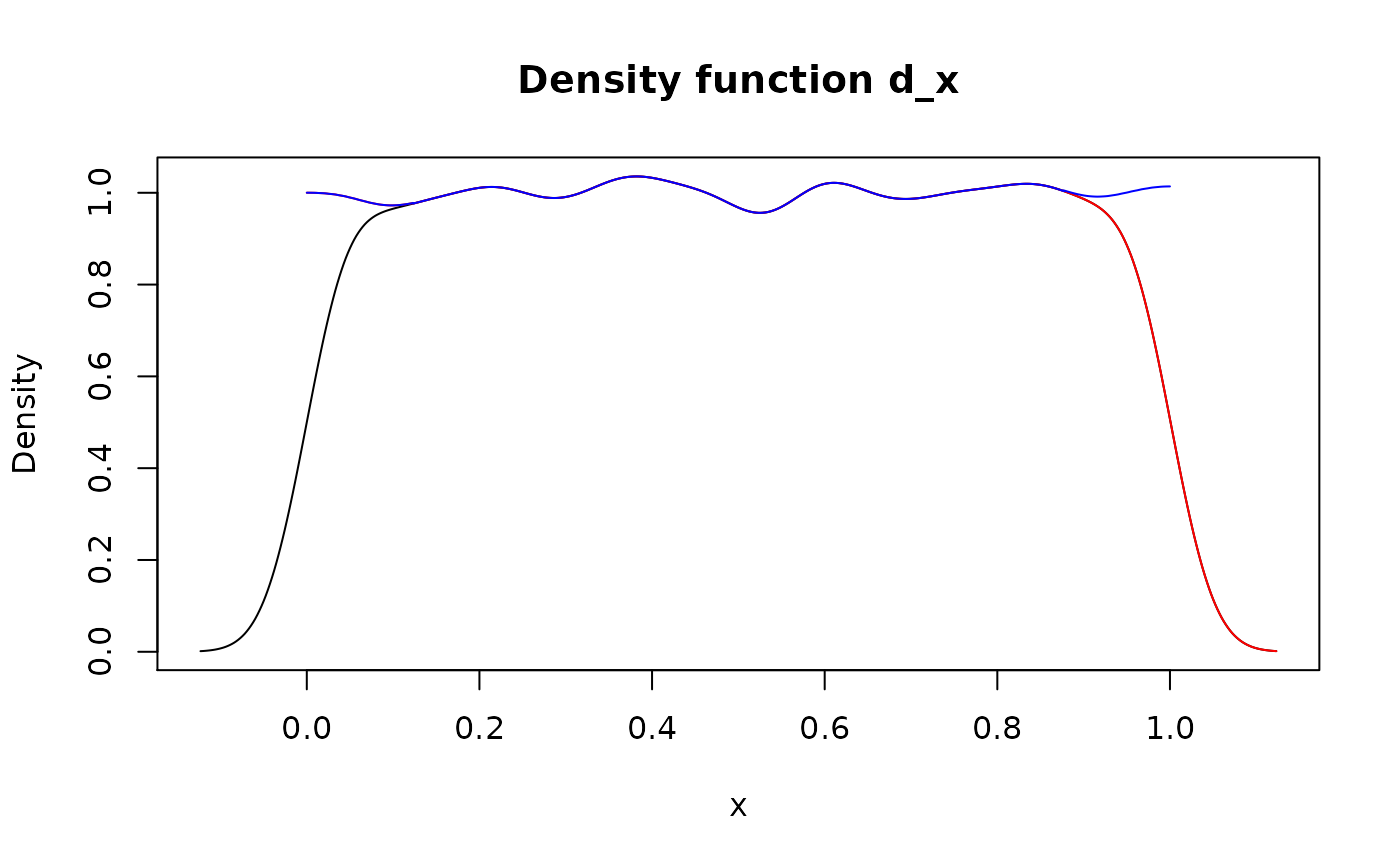# Method "trim" plot(d_norm)
lines(form_resupport(d_norm, c(-2, 1.5), "trim"), col = "blue")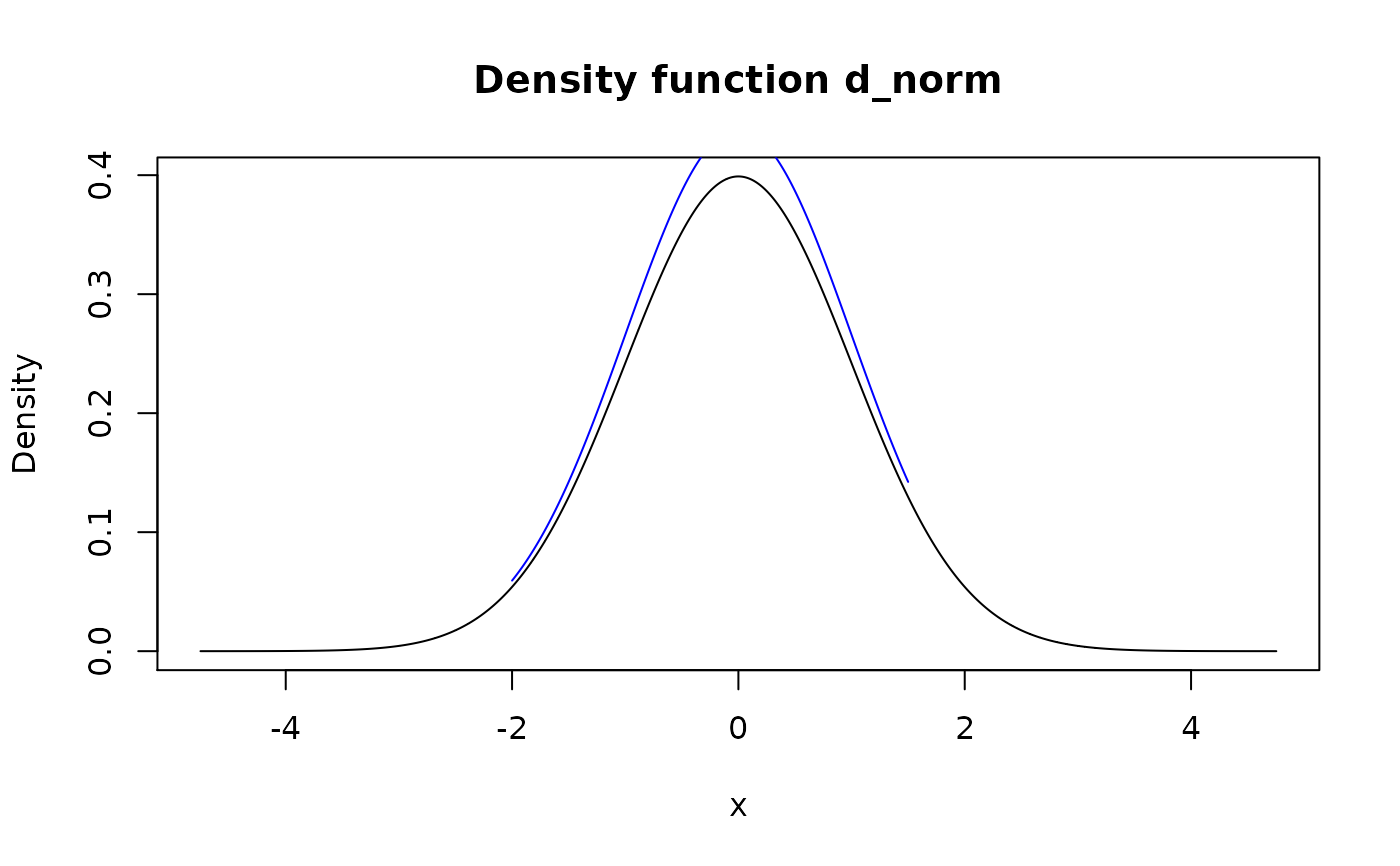# Method "winsor" plot(d_norm)
lines(form_resupport(d_norm, c(-2, 1.5), "winsor"), col = "blue")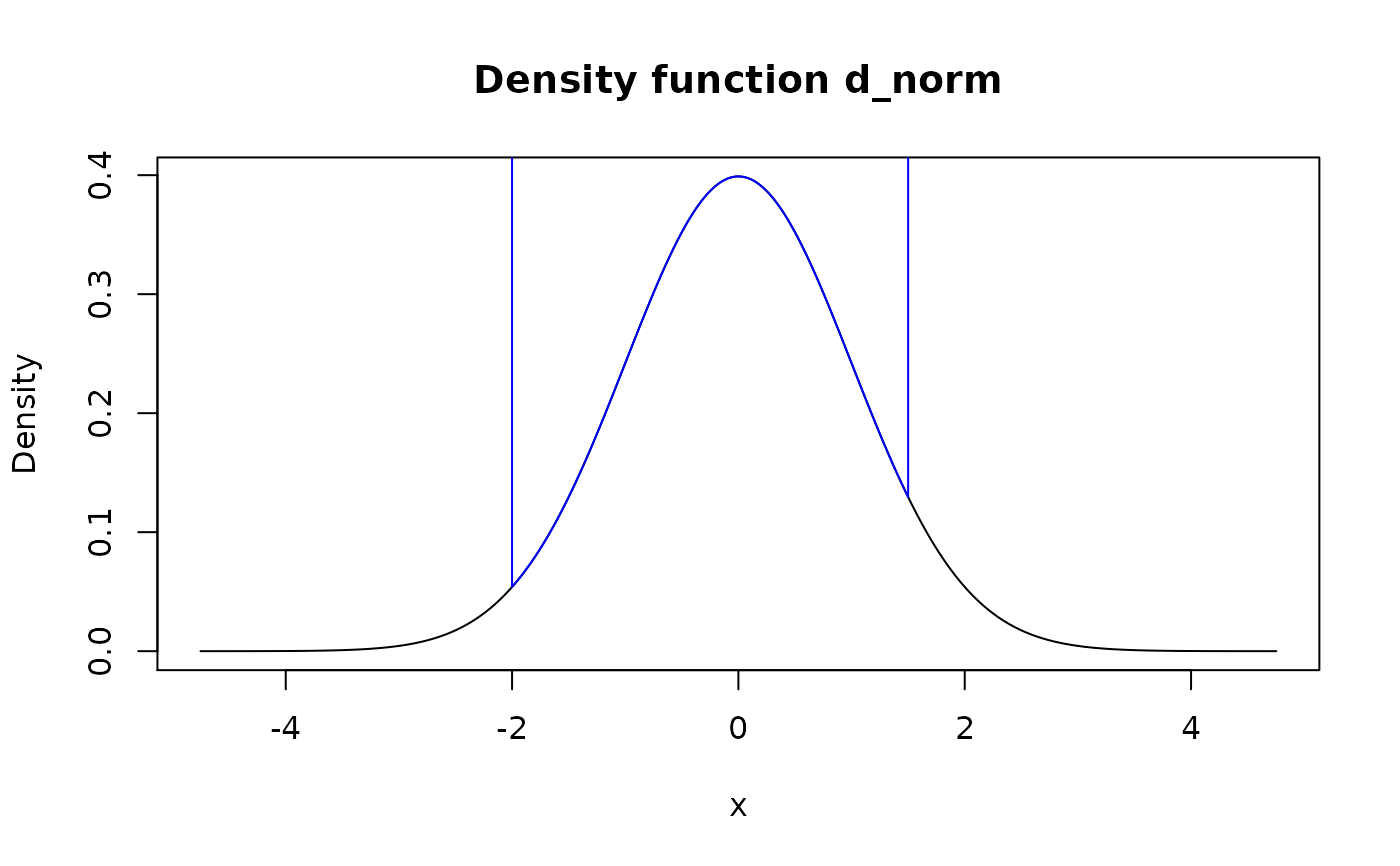# Method "linear" plot(d_norm)
lines(form_resupport(d_norm, c(-2, 1.5), "linear"), col = "blue")